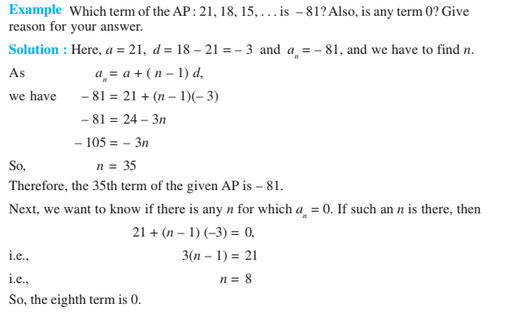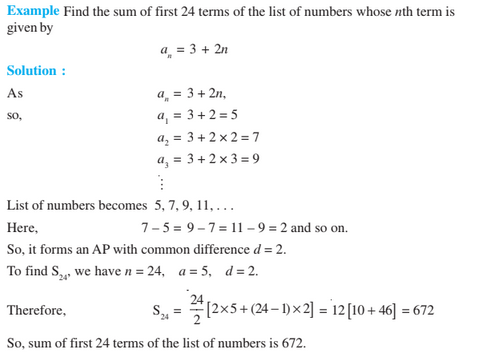# Arithmetic Progression Class 10 Notes

Arithmetic progression class 10 notes i.e. for chapter 5 provided here are an extremely crucial resource for the students studying in class 10. Chapter 5 notes are not only helpful to study the concepts in an efficient way but are also handy during the revision stage. The following topics are included in the arithmetic progression class 10 given here are –

• What is an arithmetic progression
• nth term of an AP
• Sum of n terms of an AP
• Practice Questions

## What is an Arithmetic Progression (AP)

An arithmetic progression or AP is a list of numbers in which each term differs from its preceding term by a constant number, except the first term. This fixed number is known as the common difference(d) of the A.P. This common difference can be positive, negative or even zero.

### nth term of an Arithmetic Progression (AP)

Consider the series having its first term to be ‘a’ and the common difference to be ‘d,’ then the nth term of the series is given as,

 an = a + (n−1)d

The series is given as a, a+d, a+2d,…………, a + (n−1)d### Sum of “n” Terms of an A.P

The sum of n terms of an A.P. is given as:

Sn, = n⁄2(2a + (n−1)d)

Or, Sn = n⁄2(a + a + (n−1)d)= n⁄2(a + an)

where an is the nth term of an A.P.Important Notes:

1. If “l” is the last term of the finite AP, say the nth term, then the sum of all terms of the AP
is given by n⁄2(a + l)
2. If a, b, c are in AP, then b = (a + c)⁄2 and “b” is called the arithmetic mean of a and c.

### Arithmetic Progression Class 10 Questions

1. Calculate the sum of the first 12 multiples of 13?
2. Calculate the number of terms of the AP: 9, 17, 25, . . . which must be taken to get a sum of 636?
3. Which term of the following AP is its first negative term? [AP = 121, 117, 113, . . ., ]

### Related Articles

To get more such Maths notes for class 10 and other study materials, keep visiting BYJU’S. Also, get additional study materials, question papers, and preparation tips to prepare for the class 10 board exam in a more effective way.